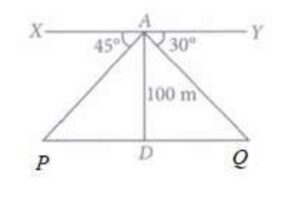Q) A boy is standing on the top of light house. He observed that boat P and boat Q are approaching the light house from opposite directions. He finds that angle of depression of boat P is 45° and angle of depression of boat Q is 30°. He also knows that height of the light house is 100 m.Based on the above information, answer the following questions.
(i) What is the measure of ∠APD?
(ii) If ∠YAQ = 30°, then ∠AQD is also 30°, Why?
(iii) Find length of PD
OR
Find length of DQ

Ans:

(i) Measure of ∠APD:

Given that ∠XAP = 45°

∴  ∠APD = 180° – 90° – 45° = 45°

Therefore, the value of ∠APD is 45°

(ii) Reason of why ∠AQD is also 30°, If ∠YAQ = 30°:

Since XY ǁ PQ and AQ cuts these parallel lines,

therefore, ∠YAQ = ∠AQD = 30° because these are alternate interior angles.

(iii) Length of PD:

In Δ APD, tan ∠APD = tan 45° =1 =PD = 100

Therefore, length of PD is 100 m

OR

(iii) Length of DQ:

In Δ AQD, tan ∠AQD = tan 30° =PD = 100√3

Therefore, length of DQ is 100√3.

Scroll to Top